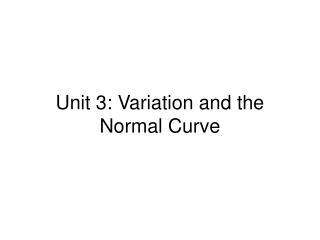DownloadDownload PresentationUnit 3: Variation and the Normal Curve

# Unit 3: Variation and the Normal Curve

Télécharger la présentation## Unit 3: Variation and the Normal Curve

- - - - - - - - - - - - - - - - - - - - - - - - - - - E N D - - - - - - - - - - - - - - - - - - - - - - - - - - -
##### Presentation Transcript

1. Unit 3: Variation and the Normal Curve

2. Review: Standard Units • “z-score” (“std units”): z = ( x – x ) / σ • the number of σ’s above average • (if negative, below average) • Ex: Data 3, 3, 5, 6, 7, 9: x = 5.5 • differences: -2.5, -2.5, -.5, .5, 1.5, 3.5 • σ = RMS of differences ≈ 2.15 • z ≈ -1.17, -1.17, -.23, .23, .70, 1.63 NOT normally distributed

3. Ex: A list of 100 numbers, already in standard units, begins -5.8, -4.3, 6.1, .2, 10.2, -3.7. Is something wrong? • They seem large -- remember, 3σ away from μ, which is ±3 in std units, is very rare • Can we check? Well, μ = 0, σ = 1, so sum of their squares should be 1 = 100/100 • But (-5.8)2 + (4.3)2 + (6.1)2 + ... is adding up to more than 100 fast • In fact, (10.2)2 alone is more than 100 • So yes, they are too big to be in std units

4. Normal table z Area(%) z Area(%) z Area(%) z Area(%) z Area(%) 0.0 0.0 0.9 63.19 1.8 92.81 2.7 99.31 3.6 99.968 0.05 3.99 0.95 65.79 1.85 93.57 2.75 99.4 3.65 99.974 0.1 7.97 1 68.27 1.9 94.26 2.8 99.49 3.7 99.978 0.15 11.92 1.05 70.63 1.95 94.88 2.85 99.56 3.75 99.982 0.2 15.85 1.1 72.87 2 95.45 2.9 99.63 3.8 99.986 0.25 19.74 1.15 74.99 2.05 95.96 2.95 99.68 3.85 99.988 0.3 23.58 1.2 76.99 2.1 96.43 3 99.73 3.9 99.99 0.35 27.37 1.25 78.87 2.15 96.84 3.05 99.771 3.95 99.992 0.4 31.08 1.3 80.64 2.2 97.22 3.1 99.806 4 99.9937 0.45 34.73 1.35 82.3 2.25 97.56 3.15 99.837 4.05 99.9949 0.5 38.29 1.4 83.85 2.3 97.86 3.2 99.863 4.1 99.9959 0.55 41.77 1.45 85.29 2.35 98.12 3.25 99.885 4.15 99.9967 0.6 45.15 1.5 86.64 2.4 98.36 3.3 99.903 4.2 99.9973 0.65 48.43 1.55 87.89 2.45 98.57 3.35 99.919 4.25 99.9979 0.7 51.61 1.6 89.04 2.5 98.76 3.4 99.933 4.3 99.9983 0.75 54.67 1.65 90.11 2.55 98.92 3.45 99.944 4.35 99.9986 0.8 57.63 1.7 91.09 2.6 99.07 3.5 99.953 4.4 99.9989 0.85 60.47 1.75 91.99 2.65 99.2 3.55 99.961 4.45 99.9991

5. Normal approx: Ex 1 • Weights in the population of a city follow the normal curve, with w = 140, σ = 30. About what % of pop weighs over 185? • In std units, 185 is (185-140)/30 = 1.5. Normal table says % > 1.5 or < -1.5 is (100-86.64)% = 13.36%. We only want right half: 13.36%/2 = 6.68% • Much too “accurate”; this is only approximation: 6.7%, or even 7%

6. Normal approx: Ex 2 • Scores on a college entrance exam follow normal curve (odd!), with x = 120 and σ = 40. • (a) About what score is the 80th %ile? • (b) About what is the IQR? • In normal table, we need z that gives percent in center, not 80%, but (80 - (100-80))% = 60%, which is z = .85. So 80th %ile of scores is [undoing std units] 120 + .85(40) ≈ 154 (b) We need z so that 50% of the data is between z and -z, and that’s z = .70. So the 3rd quartile is 120 + 40(.70), the 1st is 120 + 40(-.70), and their difference is the IQR, 2(40(.70)) = 56

7. Normal approx: Ex 3 • Data following the normal curve has avg 80 and std dev 10. • (a) What is the 15th %ile? • (b) What is the 83rd %ile? • (c) What % of data is between 85 and 95? • (d) What % of data is between 60 and 90?